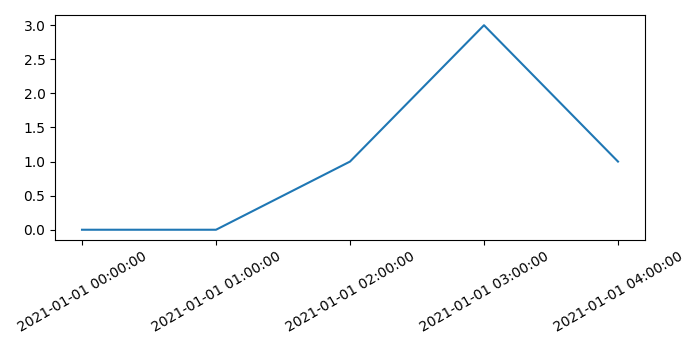# Creating a graph with date and time in axis labels with Matplotlib

To create a graph with date and time in axis labels, we can take the following steps−

• Create a figure and add a set of subplots.
• Create x and y data points using numpy.
• Set date formatter for X-axis.
• Plot x and y using plot() method.
• Set the ticks of X-axis.
• Set the date-time tick labels for X-axis, with some rotation.
• Make the plot tight layout using plt.tight_layout() method.
• To display the figure, use show() method.

## Example

from matplotlib import pyplot as plt, dates
import datetime
import numpy as np
plt.rcParams["figure.figsize"] = [7.00, 3.50]
plt.rcParams["figure.autolayout"] = True
fig, ax = plt.subplots()
x = np.array([datetime.datetime(2021, 1, 1, i, 0) for i in range(5)])
y = np.random.randint(5, size=x.shape)
ax.xaxis.set_major_formatter(dates.DateFormatter('%m-%d %H:%M'))
plt.plot(x, y)
ax.set_xticks(x)
ax.set_xticklabels(x, rotation=30, fontdict={'horizontalalignment': 'center'})
plt.show()

## Output